TestGorilla Help center

# How do I use the formula editor?

## If you need to add mathematical expressions in your question and aren't sure how, read this guide.

TestGorilla's formula editor uses LaTeX input. The good news is you don't need to know LaTeX to create beautiful mathematical expressions. We simplify the process for you!

In the question editor, click the formula icon in the rich text editing bar (highlighted in red in the picture below).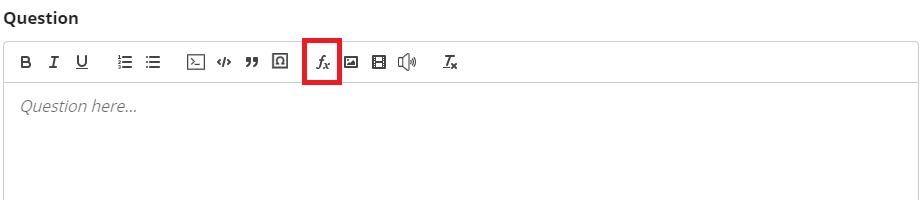This opens the formula editor (as shown below):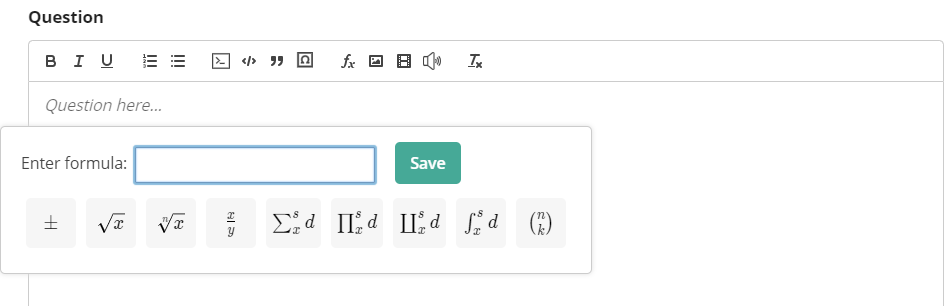Here you can enter your mathematical expression. For example, typing...

e=mc^2

... results in the following: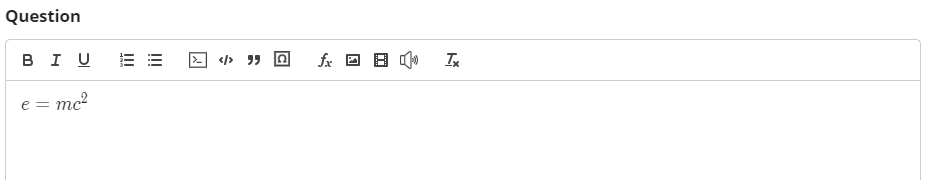To simplify your formula editing, we've added a few commonly used mathematical expressions. Simply click on one to use it in your formula.

For other expressions, you must use LaTeX codes. The following table should cover most of your needs. It's a good idea to bookmark this page so you can refer back to it as needed.

 Description Command Output plus or minus \pm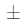multiplication (times) \times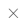multiplication (dot) \cdot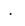division symbol \div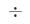simple text \text{text}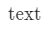infinity \infty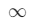dots 1,2,3,\ldots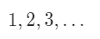dots 1+2+3+\cdots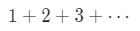fraction \frac{a}{b}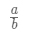square root \sqrt{x}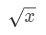nth root \sqrt[n]{x}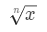exponentiation a^b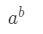subscript a_b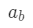absolute value |x|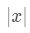natural log \ln(x)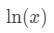logarithms \log_{a}b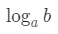exponential function e^x=\exp(x)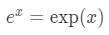deg \deg(f)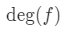degree \degree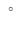circle plus \oplus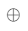circle times \otimes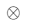not equal \ne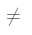less than or equal to \le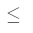greater than or equal to \ge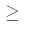approximately equal to \approx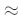vector (arrow) \vec{v}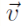derivative vector \dot{\vec{v}}vector (bold) \mathbf{v}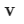unit vector (hat) \hat{k’}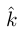norm ||\vec{v}||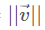element of \in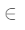subset of \subset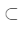subset of \subseteq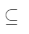contains \supset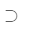contains \supseteq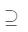union \cup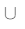intersection \cap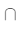big union \bigcup_{n=1}^{10}A_n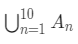big intersection \bigcap_{n=1}^{10}A_n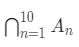empty set \emptyset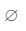power set \wp{S}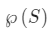minimum \min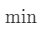maximum \max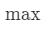closure \overline{A}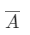derivative \frac{df}{dx}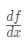derivative \f'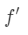partial derivative \frac{\partial f}{\partial x}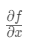limits \lim_{x\to \infty}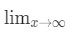summation \sum_{n=1}^{\infty}a_n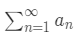product \prod_{n=1}^{\infty}a_n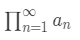integral \int_{a}^{b}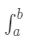double integral \iint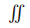triple integral \iiint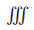If you need more symbols, you can look them up in this pdf document (there are 14,283 of them in total). Please note that not all of those symbols are available in our editor. If you're missing something, please contact us here.